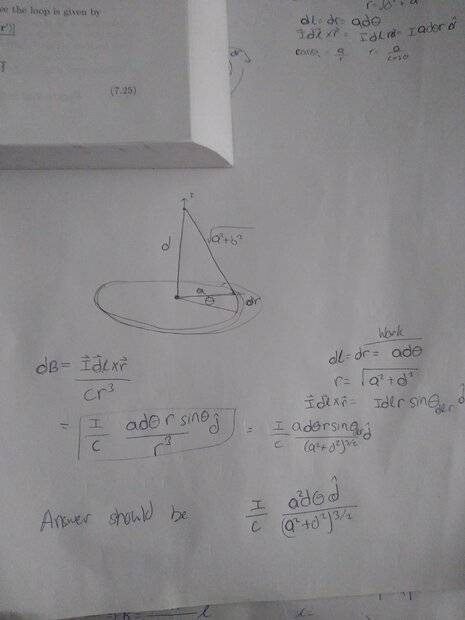# Help finding the magnetic field of a current loop

Boltzman Oscillation
Homework Statement:
Find the magnetic field at a point due to a loop of wire.
Relevant Equations:
$$db = \frac{\vec{I}\vec{dl} \times \vec{r}}{cr^3}$$
Hi all, my work is shown on the attached image. The boxed equation is what I get to but I do not understand how to go from there to what the book has. I am guessing that the problem arises when trying to solve the cross product. I understand that I will need to find the value of the sine of the angle between the arguments of the cross product but I do not see how I could do that, all I know is that the direction will be in the z axis. Any help is appreciated as always.Homework Helper
Gold Member
2022 Award
Theta is not the angle between the vectors ##\vec{d l}, \vec r##. What is the angle between them?

Boltzman Oscillation
Theta is not the angle between the vectors ##\vec l, \vec r##. What is the angle between them?
Yes, theta is not the angle between the vectors dl and r, I forgot to label the angle on the drawing. I think I can assume that angle to be close to 90° if the point is very far away but that would still not get me the answer that was provided.

Homework Helper
Gold Member
2022 Award
Yes, theta is not the angle between the vectors dl and r, I forgot to label the angle on the drawing. I think I can assume that angle to be close to 90° if the point is very far away but that would still not get me the answer that was provided.
It is not just approximately 90°, it is exactly 90°, regardless of the distance to the point. E.g. consider the point at the centre of the circle.

I'm not following how you took the z axis component of the field. Looks like you forgot to multiply by the sine of the apex half angle.

Boltzman Oscillation
It is not just approximately 90°, it is exactly 90°, regardless of the distance to the point. E.g. consider the point at the centre of the circle.

I'm not following how you took the z axis component of the field. Looks like you forgot to multiply by the sine of the apex half angle.
Im sorry but what is the apex half angle? From what I've gathered so far my current equation is
$$\frac{I*a*d \theta *r}{r^3}\vec{d}$$

I would somehow have to show that r = a which it isn't so I know somewhere a long the way I did something wrong.

I thought that by solving the cross product then I would get the z axis component. Hence:

$$\vec{I}\vec{dl} \times \vec{r} = I*dl*r*sin(\theta)\vec{z}$$

Boltzman Oscillation
It is not just approximately 90°, it is exactly 90°, regardless of the distance to the point. E.g. consider the point at the centre of the circle.

I'm not following how you took the z axis component of the field. Looks like you forgot to multiply by the sine of the apex half angle.
Ah, okay now I understand. The cross product does not show that the dB is only on the z axis so I must make that change myself. Once I took this into effect then my equation equaled that equation which I was supposed to have, many thanks!